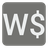Worth of Words
Elementary
RU English

Hey, are you ready for a Scrabble game party?
You have a list of words and you have to find only one that is the most valuable among them.
Rules:
The worth of each word is equivalent to the sum of letters which it consists of.
The values of the letters are as follow:
e, a, i, o, n, r, t, l, s, u = 1
d, g = 2
b, c, m, p = 3
f, h, v, w, y = 4
k = 5
j, x = 8
q, z = 10
For example, the worth of the word 'dog' is 5, because 'd' = 2, 'o' = 1 and 'g' = 2.Input: A list of words.

Output: The most valuable word.

Example:

```worth_of_words(['hi', 'quiz', 'bomb', 'president']) == 'quiz'
worth_of_words(['zero',...```
You should be an authorized user in order to see the full description and start solving this mission.